Home > CCA2 > Chapter 10 > Lesson 10.2.2 > Problem10-129

10-129.
1. Use properties of exponents to rewrite each expression below so that it involves only multiplication and exponents. Homework Help ✎

1.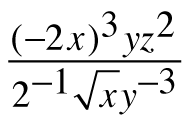2.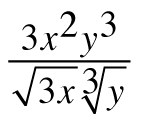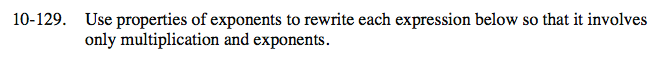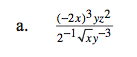$\frac{(-2)^3x^3y^1z^2}{2^{-1}x^\frac{1}{2}y^{-3}}$

$\frac{-2^3x^3y^1z^2}{2^{-1}x^\frac{1}{2}y^{-3}}$

Now apply the exponent rule for division.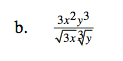$3^\frac{1}{2}x^\frac{3}{2}y^\frac{8}{3}$